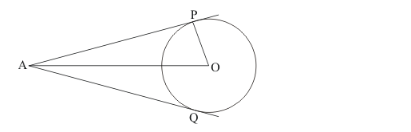# AP and PQ are tangents drawn from a point A to a circle with

Question:

AP and PQ are tangents drawn from a point A to a circle with centre O and radius 9 cm. If OA = 15 cm, then AP + AQ =

(a) 12 cm

(b) 18 cm

(c) 24 cm

(d) 36 cm

Solution:

Let us first put the given data in the form of a diagram.We know that the radius of the circle will always be perpendicular to the tangent at the point of contact. Therefore,

$O P \perp A P$

$A P^{2}=O A^{2}-O P^{2}$

$A P^{2}=15^{2}-9^{2}$

$A P^{2}=225-81$

$A P^{2}=144$

$A P=\sqrt{144}$

$A P=12$

Since tangents drawn from an external point will be equal in length,

AP = AQ

Since, AP = 12

AQ = 12

AP + AQ = 12 + 12

AP + AQ = 24

Therefore option (c) is the correct answer to this question.